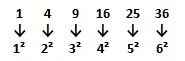1. In a certain language WEAK is coded as 9%2\$ and SKIT is coded as #\$7@, then how will WAIT be coded in the same language?
 [a] 9267 [b] 9276 [c] 92@6 [d] 927@2. Roshan is 28th from the left and Merin is 21st from the right end of row of 50 children. How many children are there between Roshan and Merin in the row?
 [a] 1 [b] 2 [c] 3 [d] 4

Number of children between Roshan and Merin in the row = 50-(28+21) = 50-49=1

3. If ASK is represented by CUM then TEN is represented by:
 [a] UDO [b] VGP [c] SCM [d] UVW4. Find the next term in the series?
1, 3, 9, 27, 81, 243, 729, ?
 [a] 2417 [b] 3287 [c] 2147 [d] 2187

1x3=3, 3x3=9, 9x3=27, 27x3=81, 81x3=243, 243x3=729, 729x3=2187

5. Next term of the sequence 1, 4, 9, 16, 25, ___ is :
 [a] 27 [b] 32 [c] 36 [d] 426. Number 4,6,8 are given. Using these numbers how many 3 digit numbers can be constructed without repeating the digits:
 [a] 4 [b] 6 [c] 7 [d] 8

3!= 1x2x3=6

7. If WALK is represented by VZKJ then TRAP is equivalent to:
 [a] USBQ [b] XBMN [c] SQZO [d] SPRQ8. If the following numbers are written in ascending order which will be the second digit of the second number?
467, 373, 411, 317, 534, 337, 587
 [a] 7 [b] 1 [c] 8 [d] 3

Ascending order of the numbers be 317, 337, 373, 411, 467, 534, 587
Second digit of the Second number=3

9. If - means x, x means +, + means ÷ and ÷ means -, what will be the value of 426+6÷70
 [a] 0 [b] 1 [c] 2 [d] 4

426+6÷70 => 426÷6-70 = 71-70=1

10. 17, 19, 20, 17, 23, 15, ___, ___
 [a] 21, 10 [b] 22, 13 [c] 26, 12 [d] 26, 13